# (GENERAL INTELLIGENCE AND REASONING)

QID : 101 -1) 9
2) 10
3) 11
4) 12
QID : 102 -1) 8
2) 10
3) 12
4) 14
QID : 103 -1) 30, 42, 01, 96, 58
2) 30, 76, 78, 04, 22
3) 66, 42, 00, 95, 58
4) 66, 77, 00, 95, 31
Correct Answer: 66, 42, 00, 95, 58
QID : 104 -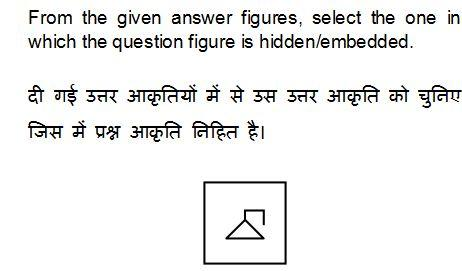1)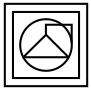2)3)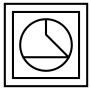4)QID : 105 -1)2)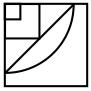3)4)QID : 106 -1)2)3)4)QID : 107 -1)2)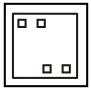3)4)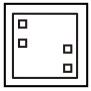QID : 108 - If '+' means '÷', '÷' means '–', '–' means '×' and '×' means '+', then
24 + 12 ÷ 6 – 4 = ?
1) – 22
2) 22
3) – 29
4) 32
QID : 109 - If 3 + 6 + 1 = 163 and 5 + 4 + 9 = 945, then 4 + 2 + 3 = ?
1) 432
2) 243
3) 234
4) 324
QID : 110 - Arrange the given words in the sequence in which they occur in the dictionary.
1. Crying
2. Crypt
3. Cooled
4. Cooking
5. Captain
1) 53421
2) 54312
3) 54132
4) 53412
QID : 111 - In the following question,select the missing number from the given alternatives.
4, 8, 16, ?, 64, 128
1) 30
2) 28
3) 24
4) 32
QID : 112 - A series is given with one term missing. Select the correct alternative from the given ones that will complete the series.
TVU, WYX, ZBA, ?
1) DEC
2) DCE
3) CED
4) CDE
QID : 113 - In the following question, select the odd word pair from the given alternatives.
1) Tall : Short
2) Above : Top
3) Laugh : Cry
4) Fat : Thin
QID : 114 - In the following question, select the odd number from the given alternatives.
1) 26
2) 64
3) 36
4) 144
QID : 115 - In the following question, select the odd letters from the given alternatives.
1) AC
2) PR
3) TU
4) JL
QID : 116 - Identify the diagram that best represents the relationship among the given classes.
Four wheelers, Jeep, Car
1)2)3)4)QID : 117 - In the following question, select the related word pair from the given alternatives.
Bird : Fly : : Fish : ?
1) Swim
2) Crawl
3) Running
4) Walking
QID : 118 - In the following question, select the related number from the given alternatives.
4 : 16 : : 9 : ?
1) 64
2) 81
3) 72
4) 90
QID : 119 - In the following question, select the related letters from the given alternatives.
ACE : BEH : : BUS : ?
1) CWV
2) CWU
3) CXV
4) CWX
QID : 120 - In the following question, select the word which cannot be formed using the letters of the given word.
HOWLINGS
1) WINS
2) HONS
3) SLIP
4) SING
QID : 121 - A walks 5 km toward west. He turns right and walks 10 km. He again turns right and walks 15 km. how far (in km) is he from his starting point?
1) 10√2
2) 10√3
3) 20
4) 10
QID : 122 - A, B, C, D and E are standing in line facing north. C stands in the middle. B and E cannot stand with C. A is not standing to the right of C. Who is standing second from left end?
1) D
2) A
3) B
4) Cannot be determined
QID : 123 - In the following question below are given some statements followed by some conclusions. Taking the given statements to be true even if they seem to be at variance from commonly known facts, read all the conclusions and then decide which of the given conclusion logically follows the given statements.
Statements:
I. All chairs are cups.
II. Some chairs are tables.
Conclusions:
I. Some tables are chairs.
II. Some tables are cups.
Options:
1) Only conclusion (I) follows.
2) Only conclusion (II) follows.
3) Both conclusion follow.
4) Neither conclusion (I) nor conclusion (II) follows.